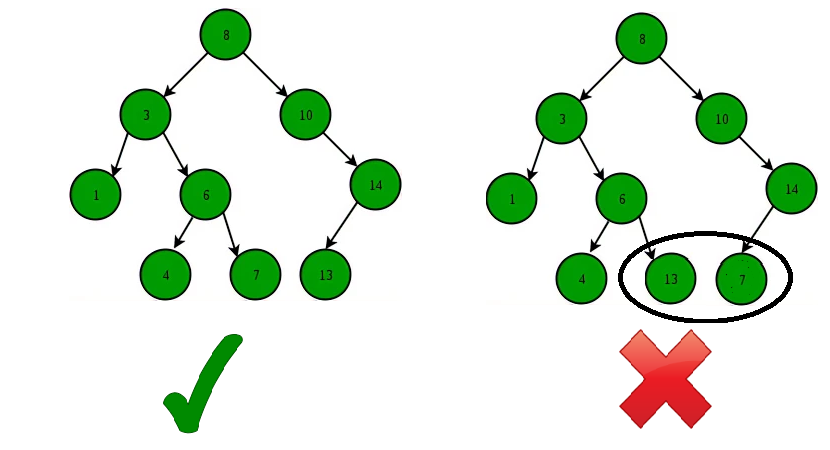GFG App
Open AppBrowser
Continue

Binary Search Tree (BST) is a data structure that is commonly used to implement efficient searching, insertion, and deletion operations. The key feature of a BST is that it is a binary tree, where each node has at most two child nodes, and the value of each node is greater than all the values in its left subtree and less than all the values in its right subtree. This means that the left subtree of a node contains values that are smaller than the node’s value, and the right subtree contains values that are larger. Due to this property, BSTs allow for efficient searching by repeatedly dividing the search space in half, which makes it an important data structure in computer science and many other fields.

• The left subtree of a node contains only nodes with keys lesser than the node’s key.
• The right subtree of a node contains only nodes with keys greater than the node’s key.
• The left and right subtree each must also be a binary search tree. There must be no duplicate nodes.Binary Search Tree – Structure

A BST supports operations like search, insert, delete, floor, ceil, greater, smaller, etc in O(h) time where h is height of the BST. To keep height less, self balancing BSTs (like AVL and Red Black Trees) are used in practice. These Self-Balancing BSTs maintain the height as O(Log n). Therefore all of the above mentioned operations become O(Log n). Together with these, BST also allows sorted order traversal of data in O(n) time.

1. A Self-Balancing Binary Search Tree is used to maintain sorted stream of data. For example, suppose we are getting online orders placed and we want to maintain the live data (in RAM) in sorted order of prices. For example, we wish to know number of items purchased at cost below a given cost at any moment. Or we wish to know number of items purchased at higher cost than given cost.
2. A Self-Balancing Binary Search Tree is used to implement doubly ended priority queue. With a Binary Heap, we can either implement a priority queue with support of extractMin() or with extractMax(). If we wish to support both the operations, we use a Self-Balancing Binary Search Tree to do both in O(Log n)
3. There are many more algorithm problems where a Self-Balancing BST is the best suited data structure, like count smaller elements on right, Smallest Greater Element on Right Side, etc.
4. One of the most common use cases of BSTs is searching for a particular element in the tree.
5.  A BST can be used to sort a large dataset. By inserting the elements of the dataset into a BST and then performing an in-order traversal, the elements will be returned in sorted order.
6. Used in Database indexing.
7. TreeMap and TreeSet are two  data structures that are internally implemented using self-balancing BSTs, more formally a Red-Black Tree.
8. BSTs can be used to implement symbol tables, which are used to store data such as variable and function names in a programming language.
My Personal Notes arrow_drop_up